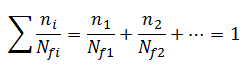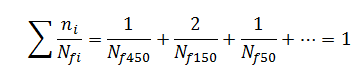you are here:/ News & Insights / Engineering Advantage Blog / How to Calculate Fatigue Life When The Load History Is Complex

# How to Calculate Fatigue Life When The Load History Is ComplexFebruary 13, 2015 By: Michael Bak

The prediction of fatigue life of structures is often challenging due to the variability and complexity of cyclic loading applied to a real structure. For example, material S-N fatigue data is generated from constant amplitude loading.  How does one calculate life in a structure whose loading represents real-world, complex variations?

The most basic fatigue loading is a constant amplitude, fully reversed load that is represented by the sine wave shown on the left of Figure 1.  Fully reversed loading means that the ratio of the minimum load over the maximum load, called the R-ratio, is equal to -1.  Since this form of loading is used for most fatigue testing, if the structure to be evaluated for fatigue life is loaded in this manner, the stress range can be directly used with the S-N curve to determine cycles to failure, as indicated in the fatigue curve on the right of Figure 1.

Figure 1: Constant Amplitude Loading Used To Predict Life From S-N Curve

## fatigue life 1.pngIf the actual fatigue loading is more complex, the cyclic content must first be extracted.  In some cases, this might be relatively straightforward if the loading consists of blocks of constant amplitude loading as shown on the left in Figure 2.  In this case, each constant amplitude loading is identified and can be evaluated separately as indicated on the right of Figure 2.

## fatigue life 2.pngOnce the number of cycles to failure for each different amplitude loading block is found, a linear damage rule is used to predict failure based on cumulative damage.  The Palmgren-Miner Rule is shown below, where n is the number of cycles in each block, and Nf is the cycles to failure for that amplitude of loading:It is interesting to note that this damage rule treats each load separately, without regard for the order of the loading or any effect of one load on another.  Although it is a very simple expression, this damage rule continues to be the standard approach for combining the effect of multiple cyclic loading in fatigue.

If the actual fatigue load history is more complex, such as in Figure 3 which could represent load data from testing or measurement, extracting the cyclic information can be challenging.

## fatigue life 3.pngA technique known as rainflow counting is commonly used to extract the cyclic data of complex loading.  The five-step procedure is summarized as follows:

1.  Determine the peaks and valleys of the stress/strain during cycling, and reorder to start from the absolute maximum (Figure 4).

2.  Visualize as draining water starting at the deepest valley (Figure 5).

3.  Measure total depth drained (stress range) and mean depth (mean stress), (Figure 5), and the number of these cycles in the load history.

4.  Continue by draining the next lowest (Figure 6) and repeat until all valleys are drained.

5.  Use the damage rule to obtain the life from all cycles.

Figure 4: Reorder to Start from Absolute Maximum

## fatigue life 4.pngFigure 5: Imagine Filling with Water and Extract Stress Range and Mean Stress

## fatigue life 5.pngFigure 6: Drain Water Starting at Lowest Valley and Repeat Cycle Extraction

## fatigue life 6.pngOnce all the cycles have been categorized, the Palmgren-Miner Rule is applied.  As an example, for this case assuming mean stress is ignored:where n is replaced with the number of actual cycles of this type in the load history, and Nf450 represents the life obtained from the S-N data for a stress range of 450.  From this equation, the number of total passes through the entire load history can be found.

The rainflow procedure can be automated so that cyclic content of complex loading can be extracted efficiently.  For example, fatigue computer codes such as nCode DesignLife will accept files of test data, or the input of multiple load steps from a static or transient finite element analysis, and use the rainflow approach to automatically extract the cyclic data.  In addition, DesignLife automates the Palmgrem-Miner Damage Rule calculation to determine number of cycles to failure, with the term cycle here defined as one pass through the entire time history.

It is generally known that stress range plays the largest role in the fatigue life, but mean stress can also have an effect.  As stated in a previous blog, mean stress effects are usually ignored in weld fatigue, but in other cases they cannot be ignored.  Stay tuned for a future blog on the effect of mean stress in fatigue!